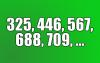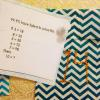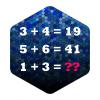BRAIN TEASERSBrain Teasers User Profile

# Filip Ginder

rank
87
points
181
See full ranking list
short ranking list
 85 Wan Ilyas 182 86 Gia Linh Luu 181 87 Filip Ginder 181 88 Brian Macpherson 176 89 Chu Đức Tuyển 174
 Find the value of the next number Look at the series (325, 446, 567, 688, 709, ...), determine the pattern, and find the value of the next number.If 3=18, 4=32, 5=50, 6=72, 7... If 3=18, 4=32, 5=50, 6=72, 7=98 then 10=?Calculate 1+3 If 3+4=19 and 5+6=41 then 1+3=?